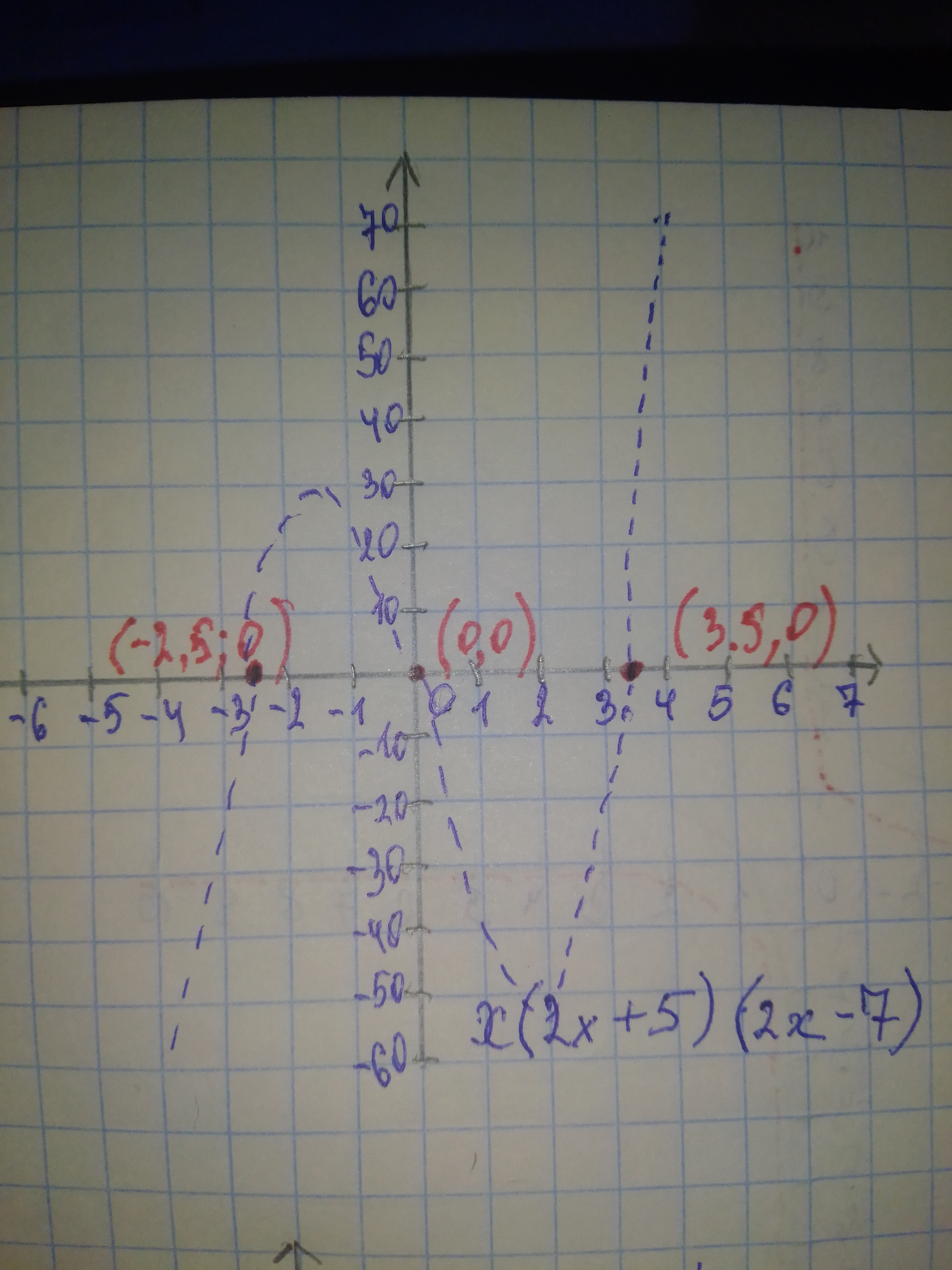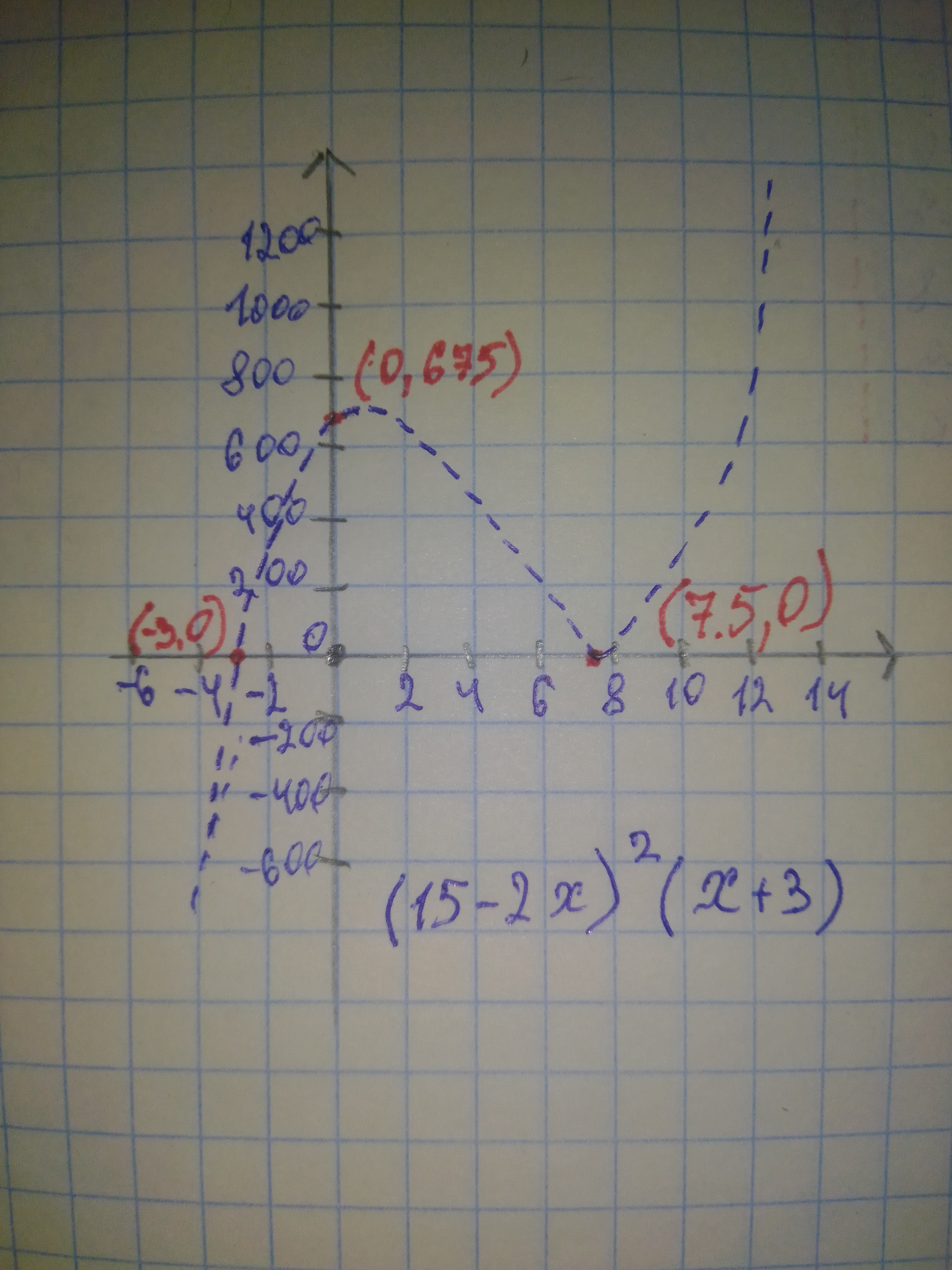# Make rough sketches of the graphs of each of the following polynomial functions. Be sure to label the x- and y- intercepts. a) y=x(2x+5)(2x-7) b) y=(15-2x)^{2}(x+3)ringearV 2020-10-23 Answered
Make rough sketches of the graphs of each of the following polynomial functions. Be sure to label the x- and y- intercepts. $a\right)y=x\left(2x+5\right)\left(2x-7\right)$ $b\right)y={\left(15-2x\right)}^{2}\left(x+3\right)$
You can still ask an expert for help

• Questions are typically answered in as fast as 30 minutes

Solve your problem for the price of one coffee

• Math expert for every subject
• Pay only if we can solve ittabuordg.